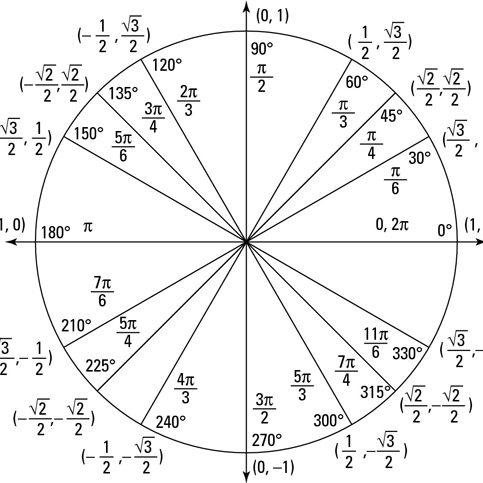## Trigonometry/Pre-Calculus

The Pre-Calculus course in Loudoun County is really a combination of Trigonometry and College Algebra.  Algebra II skills are critical - especially factoring, graphing, and solving quadratics.  Brand new material is introduced in Pre-Calculus during the Trigonometry instruction.  Heavy emphasis is placed on the unit circle, trigonometric identities, and solving trigonometric equations.  Logarithms and exponential functions are also heavily emphasized.The following concepts will be taught in the
Pre-Calculus Seminar:

1. The unit circle, principal values, and solving real world trigonometric problems
2. Graphing trig functions
3. Introduction to trig identities and solving trigonometric equations
4. Exponential and logarithmic functions and operations
5. Graphing polynomials
6. Simplifying rational expressions and graphing rational functions.

Core Math Seminars
Ashburn, VA 20147
ph: 703.201.9995
CoreMathTutoring@gmail.com